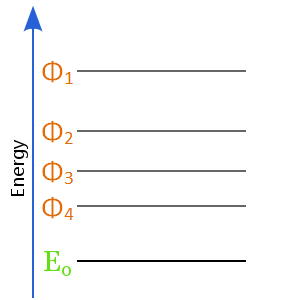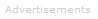# Definition of Variation Theorem

Ψ

The variation theorem is an approximation method used in quantum chemistry.

Exact, analytic solutions for the wave function, Ψ, are only available for hydrogen and hydrogenic ions. Otherwise, numerical methods of approximation must be used.Successive wave function trials, 1, then 2, then 3, then 4, result in ever better approximations to the true ground state.

The variation theorem says that no approximate wave function can have lower energy than the exact ground state energy of the system.

Another way of saying this is that the better the approximation of the wave function is, the lower its energy will be.

Trial wave functions can be tested using a computer until the one that gives the lowest energy for a system is found. Provided the mathematical form of the wave function has been chosen well, the best approximation to the true wave function will then have been found.

Making use of the variation theorem is sometimes referred to as using the perturbation method.Search the Dictionary for More Terms